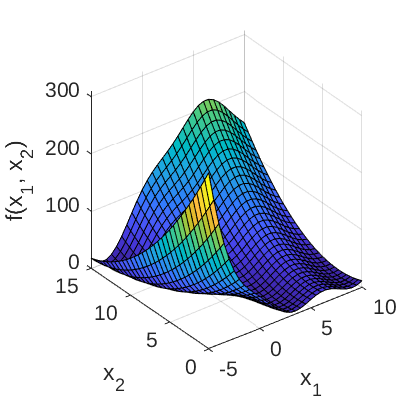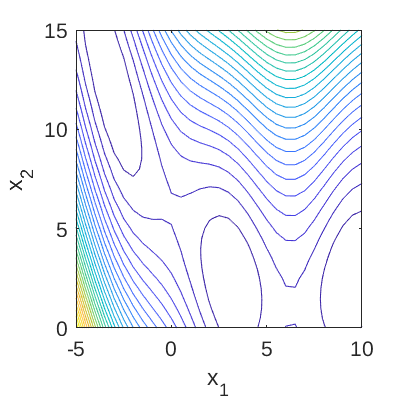# Branin Function

The Branin function is commonly used as a test function for metamodeling in computer experiments, especially in the context of optimization (Forrester et al., 2008; Picheny et al., 2012).

## Description

The analytic expression of the Branin function is given as:

f(\mathbf{x}) = a (x_2 - b x_1^2 + c x_1 - r)^2 + s (1-t) \cos{x_1} + s; \;\; x_1 \in [-5, 10], \;\; x_2 \in [0, 15],

where \mathbf{x} = \{x_1, x_2\} are input variables; and a, b, c, r, s, t are constants.

Figure 1 and 2 show the surface and contour plots of the Branin function using the default values of the constants, respectively.Figure 1: Surface plot of the Branin function.Figure 2: Contour plot of the Branin function.

## Inputs

For computer experiments, the input variables x_1, x_2 are modeled as two independent uniform random variables.

No Variable Distribution Parameters
1 x_1 Uniform x_{1,\min} = -5,
x_{1,\max} = 10
2 x_2 Uniform x_{2,\min} = 0,
x_{2,\max} = 15

## Constants

The default values for the constants are shown below.

No Constant Value
1 a 1
2 b \frac{5.1}{(2 \pi)^2}
3 c \frac{5}{\pi}
4 r 6
5 s 10
6 t \frac{1}{8\pi}

## Reference values

Using the constants above, three (identical) global minima of the function, f(\mathbf{x}^*_i) = 0.397887, are located at:

• \mathbf{x}^*_{1} = (-\pi, 12.275)
• \mathbf{x}^*_{2} = (\pi, 2.275)
• \mathbf{x}^*_{3} = (9.42478, 2.475)

## Resources

The vectorized implementation of the Branin function in MATLAB as well as the script file with the model and probabilistic inputs definitions for the function in UQLAB can be downloaded below:

uq_branin.zip (2.4 KB)

The contents of the file are:

Filename Description
uq_branin.m vectorized implementation of the Branin function
uq_Example_branin.m definitions for the model and probabilistic inputs in UQLab
LICENSE license for the function (BSD 3-Clause)

## References

• A. Forrester, A. Sóbester, and A. Keane, Engineering Design via Surrogate Modelling: A Practical Guide, West Sussex: John Wiley & Sons Ltd., 2008, pp. 196-197. DOI:10.1002/9780470770801
• V. Picheny, T. Wagner, and D. Ginsbourger, “A benchmark of Kriging-based infill criteria for noisy optimization,” HAL, hal-00658212, 2012. URL